## Понедельник 25 марта, 18-05, ауд. 203

Понедельник, 25 марта, ауд. 203. Начало в 18:05.

Докладчик: Juhani Karhumäki (University of Turku).

Тема: Variations of the Morse-Hedlund Theorem for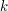-Abelian Equivalence.

### Abstract

In this talk we investigate local to global phenomena for a new family of complexity functions of infinite words indexed by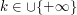wheredemotes the set of positive integers. Two finite wordsandin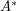are said to be-Abelian equivalent if for all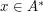of length less than or equal to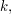the number of occurrences ofinis equal to the number of occurrences ofinThis defines a family of equivalence relations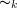on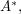bridging the gap between the usual notion of Abelian equivalence (when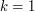) and equality (when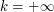). Given an infinite word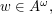we consider the associated complexity function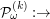which counts the number of-Abelian equivalence classes of factors ofof lengthAs a whole, these complexity functions have a number of common features: Each gives a characterization of periodicity in the context of bi-infinite words, and each can be used to characterize Sturmian words in the framework of aperiodic one-sided infinite words. Nevertheless, they also exhibit a number of striking differences, the study of which is one of the main topics of our talk. The talk is based on the join work with Aleksi Saarela and Luca Q. Zamboni.